# How to Use SUMIF Function in Excel

As the name suggests, the SUMIF function is used in Excel formulas to sum up the values in a range on a given condition.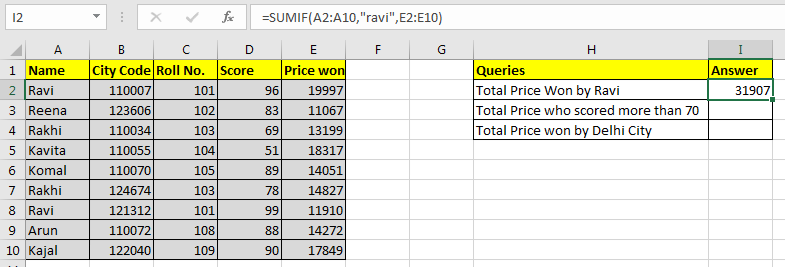Generic Excel SUMIF Formula:

=SUMIF(condition_range,condition,sum range)

Let's jump into an example. But theory…, Ah! We will cover it later.

## Use SUMIF To Sum Values On One Condition

For this example I have prepared this data.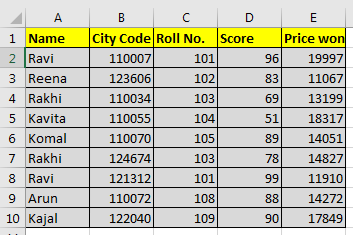Based on this data we need to answer these questions: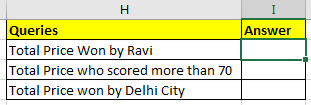## SUMIF with Text Condition

We need to tell the sum of the price won by Ravi.
So our condition range will be name range and that is A2:A10.
Our condition is Ravi and
Sum range is E2:E10.

So in cell I2 we will write:

=SUMIF(A2:A10,"ravi",E2:E10)

Note that ravi is in double quotes. Text conditions are always written in double quotes. This is not the case with numbers.
Note that ravi is written in all smalls. Since SUMIF is not case sensitive, hence it doesn’t matter.

The above SUMIF formula will return 31907 as shown in the image below.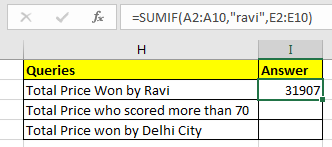## SUMIF with Logical Operators

For the second question, our condition range will be D2:D10.
Condition is >70 and
The sum range is same as before.

=SUMIF(D2:D10,">70",E2:E10)

The above SUMIF formula will return 103973 as shown in the image below.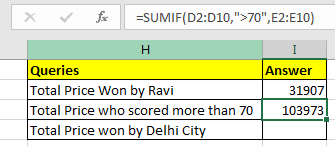## SUMIF with Wild Card Operators

In the third question, our condition is Delhi. But we don’t have a city column. Hmmm… So what do we have? Aha! City code. This will work.
We know that all Delhi city codes start from 1100. City codes are in 6 digits. So we know it is 1100??. “?” operator is used when we know number of characters but don’t know the character. As here we know that there are two more numbers after 1100. They can be anything, so we used “?”. If didn’t know the number of characters, we would use “*”.
Remember wild card operators only work with text values. Hence you need to convert city code into text.

You can concatenate numbers with “” to make them text value.

(formula to convert number into text) = number & “” or =CONCATENATE(number,””)

Now in cell I2 write this formula

=SUMIF(B2:B10,"1100??",E2:E10)

This will return the sum of the price whose city code starts with 1100. In our example it is 79836.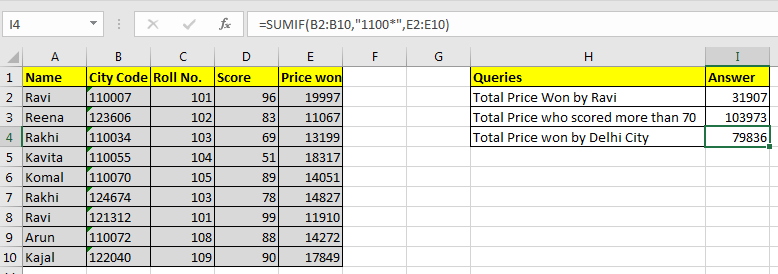Pro Notes:

• If your sum range and condition range are the same, you can omit the sum_range variable in SUMIF function. =SUMIF(E2:E10,">15000") and =SUMIF(E2:E10,">15000",E2:E10) will produce same result, 56163.
• Text values are encapsulated in double quotes, but numbers do not. =SUMIF(C2:C10,103,E2:E10) this will work fine and will return 28026. However while working with logical operators you need to use double quotes. Like our example =SUMIF(D2:D10,">70",E2:E10)
• It can check only one condition. For multiple conditions we use SUMIFS function in Excel.

Question: I have Sales data that contains Month, Region & Sales in value. Now, what I want in Excel is to calculate the following:

• Sales in a specific month for all the regions
• Total Sales except a particular month
• Sales for a specific month & region

Following is the snapshot of data: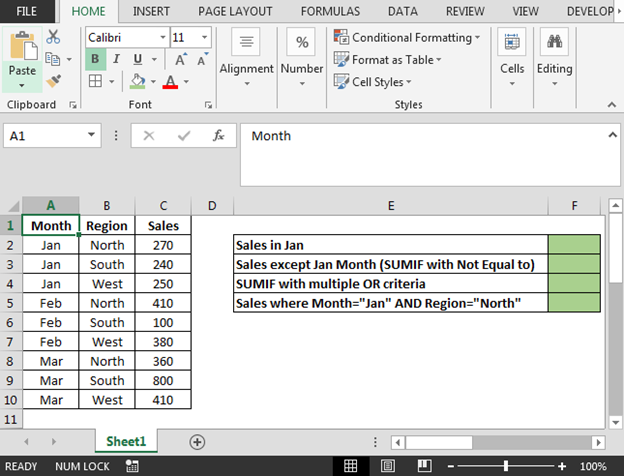• To calculate Sales in a Jan month for all the regions; the formula in cell F2 is
• =SUMIF(A2:A10,"Jan",C2:C10)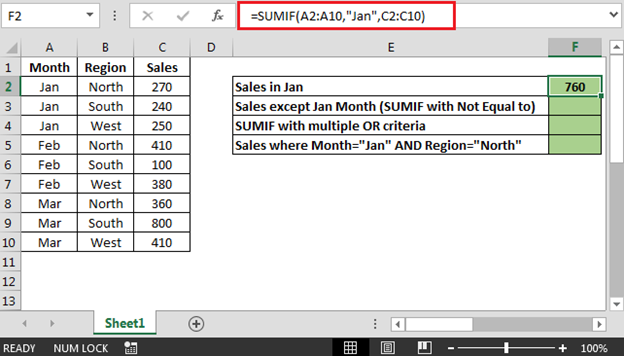• To get Total Sales except a Jan month i.e. we can use SUMIF not equal to sign (<>); the formula in cell F3 is
• =SUMIF(A2:A10,"<>Jan",C2:C10)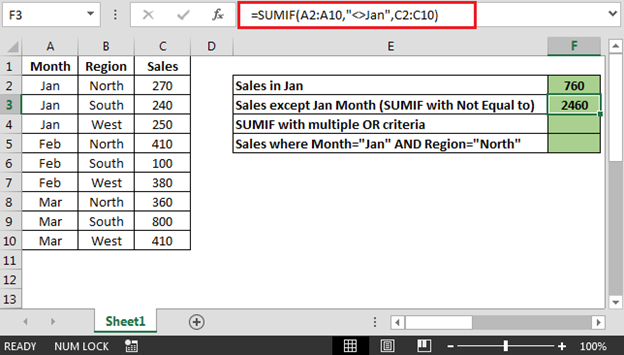• To get Total Sales using OR criteria; the formula in cell F4 is
• =SUMIF(A2:A10,"Feb",C2:C10)+SUMIF(A2:A10,"Mar",C2:C10)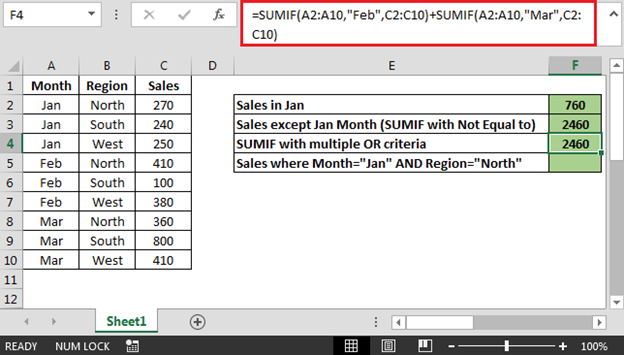• To get Sales for a specific month & region; the formula in cell F5 is
• {=SUM((A2:A10="Jan")*(B2:B10="North")*C2:C10)}

Note: This is an array formula. Use CTRL + SHIFT + ENTER keys together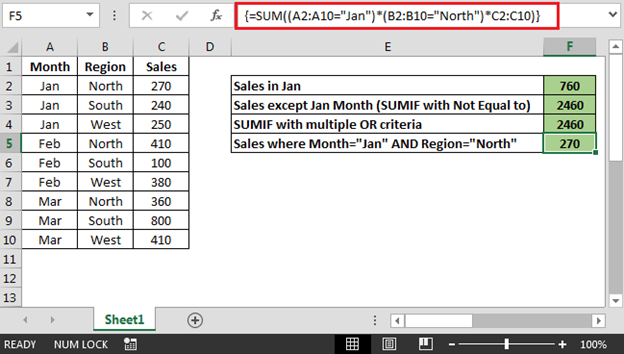Related Articles

3 SUMIF with Or Formulas

How to Use SUMIFS Function in Excel

SUMIFS using AND-OR logic

SUMIF with non-blank cells

SUMIFS with dates in Excel

## Users are saying about us...

1.How do I work out in Excel, the cost of work that has taken say, from 9:00 till 10:oo is it £20, then till 11:30 is it £28 and then till 12:45 is it £45

Terms and Conditions of use

The applications/code on this site are distributed as is and without warranties or liability. In no event shall the owner of the copyrights, or the authors of the applications/code be liable for any loss of profit, any problems or any damage resulting from the use or evaluation of the applications/code.